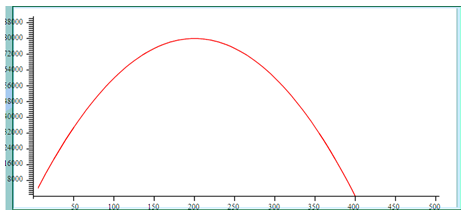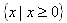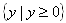Area Problems
Example Continued

Step 3. Rewrite the equation using x and y variables and graph using a graphing calculator.• Since the inputs are based on width, the domain is all real numbers greater than or equal to 0;.
• Since the outputs are based on area, the range is all real numbers greater than or equal to 0;.